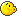## A plus B... again

Given two integers A and B (of no more than 100000 digits each), find their exact sum.

Point Value: 15
Time Limit: 3.00s
Memory Limit: 16M

Problem Types: [Show]

Languages Allowed:
C++03, PAS, C, ASM, C#, C++11

<
1
2
3
>

• (1/1)
1 + 10
1 + 9
99 + 1
-99 - 1
100 - 1
1 - 11
123456789 + 987654321

Good luck!

• (0/2)
cause that's too easy

• (0/0)
My code WAs here, but ACs on DMOJ. Any reason why?
What happened to the test cases

• (1/0)
Read the problem statement. It's only one sentence.

• (0/2)
in java, just use long insted of int

• (4/3)
It's not like it makes it any easier, we still have to use string manipulation because java variables can't store such big integers.

• (11/2)
Java has a built-in BigInteger class. A sample Java solution for this problem is as follows:

``import java.util.Scanner; import java.math.BigInteger;  public class aplusb2 {     public static void main(String[] foo) {         Scanner in = new Scanner(System.in);         System.out.println((new BigInteger(in.next())).add(new BigInteger(in.next())));     } }``

• (0/0)
What's the difference between
public static void main(String[] args)
and
public static void main(String[] foo)?

• (2/2)
If that's a sample Java solution for this problem, then why can't we submit it in JAVA? I mean, if you say that that is a way to solve it, then it means that there is a way to solve it in JAVA

• (0/1)
what is the point of this problem?
isnt it just like the first one?

• (0/0)
64 bit integers cannot hold numbers of 100000 digits (i.e., of size 10^100000)

• (0/2)
lol

• (1/1)

• (1/1)
yes, otherwise the problem would be trivial.

• (2/2)
The program is not working!
Is there a catch? I have used the same program as the
first A + B.
For Some reason it no work!
Its very Simple!

_________________

``var a,b:integer; begin     readln (a);     readln (b);     writeln (a+b); end.``

nothing to see here• (10/2)
http://wiki.freepascal.org/Integer

Integers only have a capacity of -231 to 231 -1 which is 10 digits long while this problem has input that may be up to 100 000 digits each. So you'll have to find another method to solve this. For future reference don't post your code in the comments and please refer to this before posting a question.